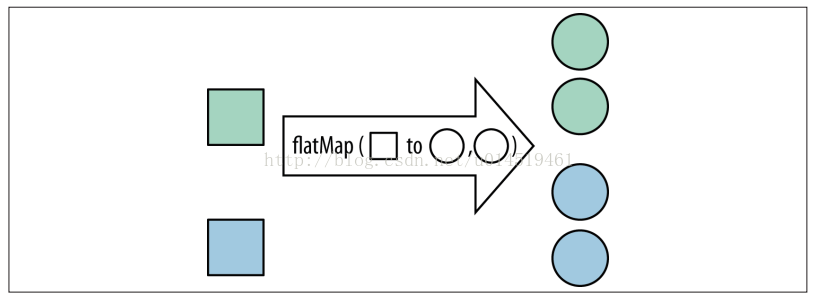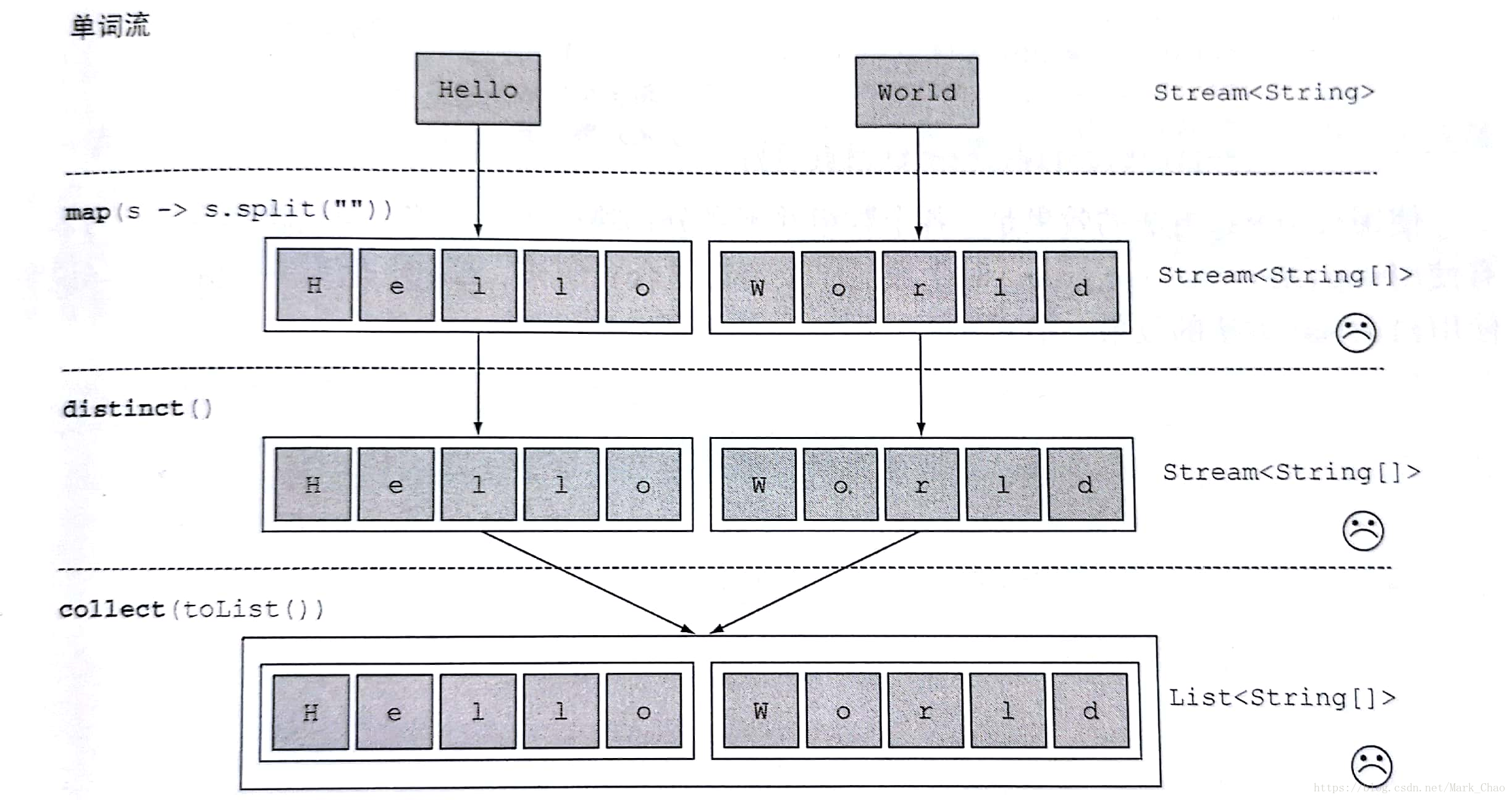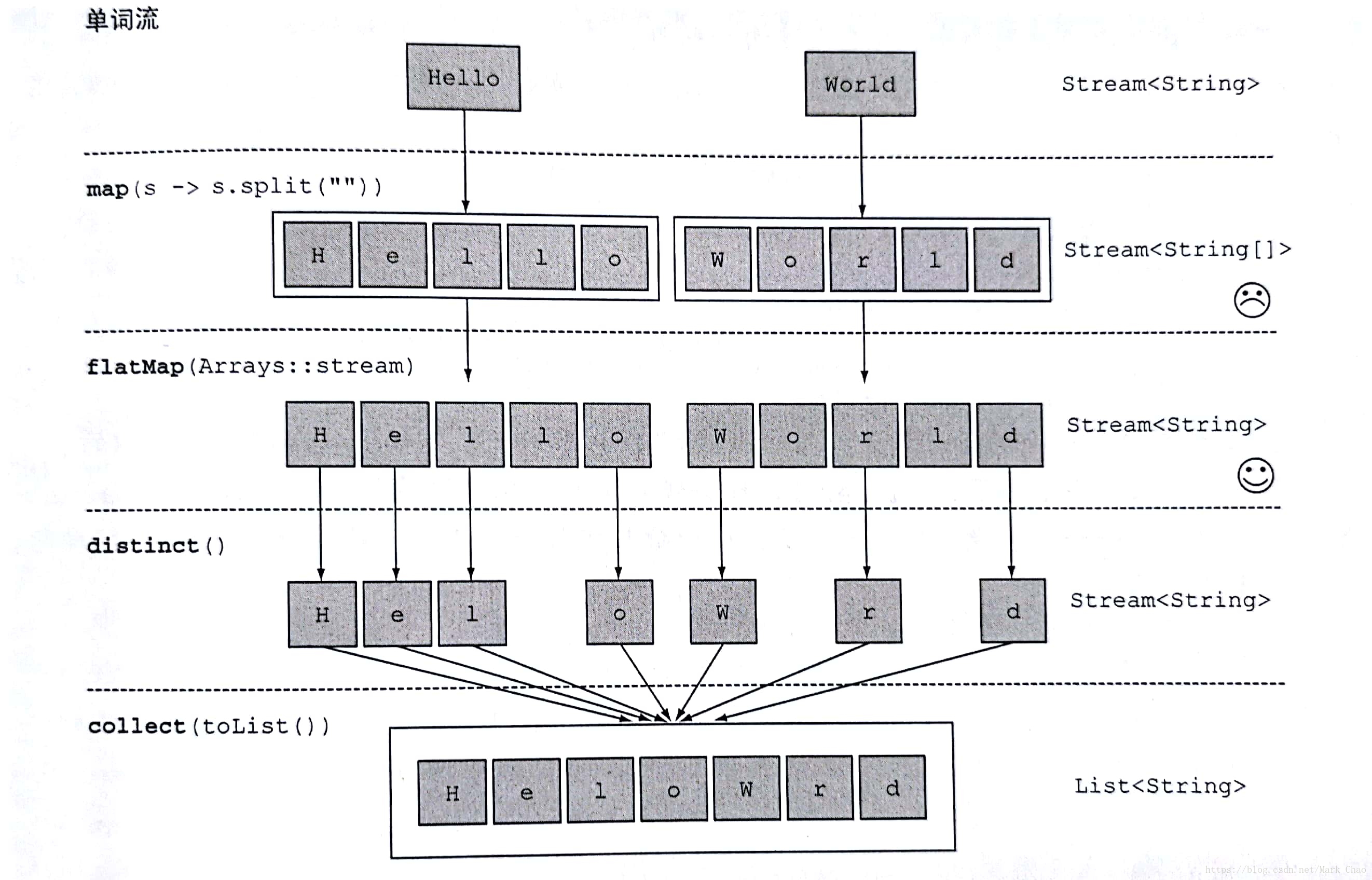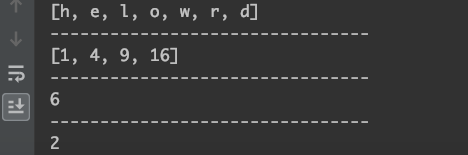• 如果您觉得本站非常有看点，那么赶紧使用Ctrl+D 收藏吧

# java8 stream flatMap流的扁平化操作

1次浏览

2种操作：

1.intermediate  operation 中间操作：中间操作的结果是刻画、描述了一个Stream，并没有产生一个新集合，这种操作也叫做惰性求值方法。

2.terminal operation 终止操作：最终会从Stream中得到值。

flatMap 中间操作:``````	@Test
public void flapMapTest() {
List<Integer> list = (List<Integer>) Stream.of(Arrays.asList(1, 2, 3, 4, 5, 6), Arrays.asList(8, 9, 10, 11, 12))
.flatMap(test -> test.stream()).collect(Collectors.toList());

for (int i = 0, length = list.size(); i < length; i++) {
System.out.println(list.get(i));
}

}``````

flatMap的用法和含义住要通过一个案例来讲解，

``````
String[] words = new String[]{"Hello","World"};

List<String[]> a = Arrays.stream(words)

.map(word -> word.split(""))

.distinct()

.collect(toList());

a.forEach(System.out::print);``````

代码输出为：[Ljava.lang.String;@12edcd21[Ljava.lang.String;@34c45dca

（返回一个包含两个String[]的list）

这个实现方式是由问题的，传递给map方法的lambda为每个单词生成了一个String[](String列表)。因此，map返回的流实际上是Stream<String[]> 类型的。你真正想要的是用Stream<String>来表示一个字符串。

下方图是上方代码stream的运行流程``````String[] words = new String[]{"Hello","World"};

List<String> a = Arrays.stream(words)

.map(word -> word.split(""))

.flatMap(Arrays::stream)

.distinct()

.collect(toList());

a.forEach(System.out::print);``````

使用flatMap方法的效果是，各个数组并不是分别映射一个流，而是映射成流的内容，所有使用map(Array::stream)时生成的单个流被合并起来，即扁平化为一个流。

下图是运用flatMap的stream运行流程，示例：

``````
import java.util.ArrayList;
import java.util.Arrays;
import java.util.List;
import java.util.stream.Collectors;

public class FlatMap {
public static void main(String[] args) {
//扁平化流
//找出数组中唯一的字符
String[] strArray = {"hello", "world"};

//具体实现
List<String> res = Arrays.stream(strArray)
.map(w -> w.split(""))
.flatMap(Arrays::stream)
.distinct()
.collect(Collectors.toList());
System.out.println(res);

//TODO 案例
System.out.println("--------------------------------");
//Demo1:给定数组，返回数组平方和(直接使用映射)
//[1,2,3,4]=>[1,4,9,16]
Integer[] nums1 = {1, 2, 3, 4};
List<Integer> nums1List = Arrays.asList(nums1);
List<Integer> res1 = nums1List.stream().map(i -> i * i).collect(Collectors.toList());
System.out.println(res1);

System.out.println("--------------------------------");
//Demo2:给定两数组，返回数组对
//[1,2,3],[3,4]=>[1,3],[1,4],[2,3],[2,4],[3,3],[3,4]
Integer[] nums2 = {1, 2, 3};
Integer[] nums3 = {3, 4};
List<Integer> nums2List = Arrays.asList(nums2);
List<Integer> nums3List = Arrays.asList(nums3);

//使用2个map嵌套过滤
List<int[]> res2 = nums2List.stream().flatMap(i -> nums3List.stream().map(j -> new int[]{i, j})).collect(Collectors.toList());
System.out.println(res2.size());

System.out.println("--------------------------------");
//Demo3:针对Demo2和Demo1组合返回总和能被3整除的数对
//(2,4)和(3,3)是满足条件的
List<int[]> res3 = nums2List.stream().flatMap(i -> nums3List.stream().filter(j -> (i + j) % 3 == 0).map(j -> new int[]{i, j})).collect(Collectors.toList());
System.out.println(res3.size());

}
}``````